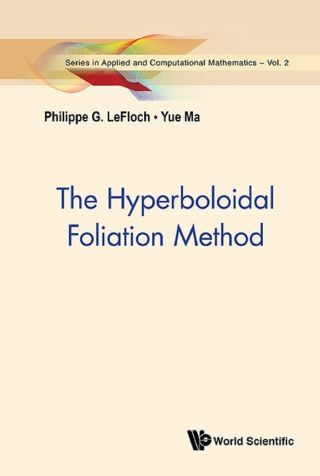## Hyperboloidal Foliation Method, The• Description
• Author
• Info
• Reviews

### Description

The “Hyperboloidal Foliation Method” introduced in this monograph is based on a (3 + 1) foliation of Minkowski spacetime by hyperboloidal hypersurfaces. This method allows the authors to establish global-in-time existence results for systems of nonlinear wave equations posed on a curved spacetime. It also allows to encompass the wave equation and the Klein-Gordon equation in a unified framework and, consequently, to establish a well-posedness theory for a broad class of systems of nonlinear wave-Klein-Gordon equations. This book requires certain natural (null) conditions on nonlinear interactions, which are much less restrictive that the ones assumed in the existing literature. This theory applies to systems arising in mathematical physics involving a massive scalar field, such as the Dirac-Klein-Gordon systems.

Contents:
• Introduction
• The Hyperboloidal Foliation and the Bootstrap Strategy
• Decompositions and Estimates for the Commutators
• The Null Structure in the Semi-Hyperboloidal Frame
• Sobolev and Hardy Inequalities on Hyperboloids
• Revisiting Scalar Wave Equations
• Fundamental L and L2 Estimates
• Second-Order Dervatives of the Wave Components
• Null Forms and Decay in Time
• L2 Estimate on the Interaction Terms
• The Local Well-Posedness Theory
• Bibliography

Readership: Graduate students and researchers in analysis and differential equations. This book could serve as part of graduate course on nonlinear wave equations.